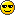# Homework 1##Homework 1

Hwk 1 is about MCMC and Page Rank and I am in charge of it. Please send your copy to me (alberto.rosso74@gmail.com) and I will send you an e-mail to confirm the reception.

Have Fun!

Alberto

##Re: Homework 1

Deadline of Hwk 1 : October 18, 2018. 1 pm

##Re: Homework 1

Thank you Alberto, very nice initiative! I hope that this forum will foster interesting and useful discussions.##Re: Homework 1

Good Evening Dr Rosso

A question about the homework: in the definition of autocorrelations functions,
1- what does the subscript "i" mean ?
2- should we answer this question with the first data (sampling according to exp(-V)) or the bunched data just obtained ?

Thank you

##Autocorrelation function

Dear remyk,

1- In Q3 the subscript "i" means the ith element of your long list of data. In principle the autocorelation function depends both on i and n. However we remark that
"Here we consider long Markov chains so that both Cr(n) and Cx(n) are independent of i (stationary limit)."

2- you should use the original data not the bunched ones.

Good night,

Alberto

##Re: Homework 1

Thanks a lot Admin ;-)

##Re: Homework 1

Dear Pr. Rosso,

I have a question on the meaning of the mean value of the product for the correlation function. Indeed, the index i is fixed outside the mean value.
1- In Q3 the subscript "i" means the ith element of your long list of data. In principle the autocorelation function depends both on i and n. However we remark that
"Here we consider long Markov chains so that both Cr(n) and Cx(n) are independent of i (stationary limit)."

Could you explain what does this average mean please ?

##Re: Homework 1

In the stationary limit C(n) is the average value of two elements that have a distance n in the Markov chain. Thus, you can obtain C(n) even from a single (but long) list of data, by averaging over i and keeping fixed n.

Best Regards,

Alberto

##Re: Homework 1

Good evening Dr Rosso

2 new questions:

- for auto correlation function, is it normal to obtain correlation times that are slightly different from the estimations with bunching ? (however the factor of "discrepancy" is the same for t_r and t_x between the two methods). After having discussed about that between us, it seems to be a usual observation.

- In question Q9a, we assume that once the Surfer jumps in a dead end, it selects a random page of the graph. Can he select any page including this dead end or not ?

Thank you

##Re: Homework 1

- The most important thing is that the estimation of the error obtained with the two methods is the same. If the definition of the correlation time is slightly different for the two methods, your estimation can be slightly different.
- "Can he select any page including this dead end or not". Both strategies are OK to solve the dead end problem.

Have a nice Sunday,

Alberto

##Re: Homework 1

Hi Dr. Rosso,

A couple of questions:

1. For calculating the correlation functions, can I replace the ensemble average with the time average by running a single Markov Chain?

2. I am not sure what to do in Q7 of the page rank part. It says "Show the validity of the PageRank equation written by Page and Brin" - Show how ? By an analytical calculation that establishes that the given equation gives the top Eigenvector of the Transition matrix?

3. In Q8, for the PageRank function - do I just find the top Eigenvector or somehow otherwise calculate the pagerank ? Also for the next part of the same question - can I use any starting point for the surfer and just repeatedly apply the transition matrix?

Thank you.

##Re: Homework 1

Hello,

1. Yes.
2. Yes by an analytical calculation
3. Yes you have to provide the ranking of the different web page. Yes you can.

Alberto

##Re: Homework 1